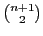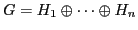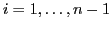# Topological, Geometric and Algebraic Graph Theory

### Monday January 25

9:00-9:30
Cristina Dalfó Simó (Universitat Politècnica de Catalunya)
On almost distance-regular graphs

Distance-regular graphs have been a key concept in Algebraic Combinatorics and have given place to several generalizations, such as association schemes and almost distance-regular graphs, which are the topic of this talk. Roughly speaking, almost distance-regular graphs have some kind of regularity that is characteristic of distance-regular graphs. An example is the concept of m-walk regularity, which is a generalization of walk-regularity, the latter defined in terms of the invariance of the number of l-walks between vertices at a given distance at most m. Other concepts are those of m-partially distance-polynomial and m-partially distance-regular graphs, characterized by the existence of polynomials with a certain degree in the adjacency matrix of the graphs, which give its corresponding distance matrices. In this talk, we give some algebraic and combinatorial characterizations of such almost distance-regular graphs, based on the so-called local spectrum and predistance polynomials of the graph. The main results can be seen as a generalization for almost distance-regular graphs of the spectral excess theorem for distance-regular graphs, which provides a quasi-spectral characterization in terms of the excess (number of vertices at maximum distance) of each of its vertices.

(joint work with E.R. van Dam, M.A. Fiol, E. Garriga, and B.L. Gorissen).

9:30-10:00
Marc Cámara Vallejo (Universitat Politècnica de Catalunya)
Pseudo-distance-regular graphs around a set and the local spectrum of its subconstituents

Pseudo-distance-regularity around a set of vertices generalizes the notion of completely regular code to non-necessary regular graphs. In this talk we will study some quasi spectral characterizations of pseudo-distance-regularity around a set of vertices. These characterizations are obtained through the study of the local spectrum of a set of vertices, which play a similar role to that of the spectrum of the whole graph when it is seen from that set of vertices. We will also discuss the relation between the local spectrum of a set of vertices and that of its subconstituents, paying special attention to its antipodal set.

10:00-10:30
Roman Nedela (Matej Bel University, Banská Bystrica)
Skew-morphisms of groups and regular maps

Skew-morphism of a group is a generalization of a group automorphism. Skew-morphisms were investigated in connection of regular embeddings of graphs. In the present talk we survey some results on this interesting relationship. Further, we present some results on skew-morphisms of cyclic groups.

11:00-11:30
Daniel Pellcer (University of Ljubljana)
Vertex-transitive maps with Schläfli type 3,7

When classifying maps with certain degree of symmetry on surfaces of small Euler characteristic the Hurwitz bounds play an important role. The equivelar maps with highest Hurwitz bounds are those whose vertices are 3-valent and whose faces are heptagons, as well as their duals. If these maps are classified, the computer time to compute the remaining equivelar maps reduces considerably. In this talk we present a procedure to classify all 3-valent vertex-transitive maps with heptagonal faces and their duals by means of smaller regular or 2-orbit maps.

11:30-12:00
Tomaž Pisanski (University of Ljubljana)
Applications of symmetries of combinatorial maps

In 1980 combinatorial maps were introduced by A. Vince in his thesis. They are a useful tool for investigation of various combinatorial structures such as maps or abstract polytopes. Each combinatorial map admits a unique quotient "type graph". Using elementary methods related to type graphs we derive the 14 types of edge-transitive maps first studied by J. Graver and M. Watkins in 1997. Applications to abstract polytopes are also mentioned.

12:00-12:30
Jozef Siran (Comenius University, Bratislava)
Residual finiteness and symmetric maps

A group is residually finite if for any of its non-identity elements the group contains a subgroup of finite index avoiding the chosen element. Among the important classes of residually finite groups are automorphism groups of tessellations of the infinite plane. Since any map on some surface, and in particular any `highly symmetric' map, is a quotient of such a tessellation, residual finiteness may help proving theorems for highly symmetric maps. We outline the corresponding theory and give illustrative examples.

14:00-14:25
Ján Karabáš (Matej Bel University, Banská Bystrica)
Archimedean solids of higher genera

We will deal with vertex-transitive and polyhedral maps embeded into orientable surfaces of higher genera. These maps naturally generalise the spherical maps associated with classical Archimedean solids. Therefore we call them Archimedean maps. The main idea is based on the fact that each Archimedean map projects onto one-vertex (or two-vertex) quotient map. For given genus g there are just finitely many quotients and all Archimedean maps of genus g can be reconstructed from these quotients. We will also show the sketch of method of construction of unoriented "Archimedean maps" of higher genera.

14:25-14:50
Pavel Valtr (Charles University, Prague)
Coding and Counting Arrangements of Pseudolines

Arrangements of lines and pseudolines are important and appealing objects for research in discrete and computational geometry. We show that there are at most 20.66 n2 simple arrangements of n pseudolines in the plane. This improves on previous work by Knuth who proved an upper bound ofin 1992 and S. Felsner who obtained 20.69 n2 in 1997. The argument uses surprisingly little geometry. The main ingredient is a lemma that was already central to the argument given by Knuth.

Joint work with Stefan Felsner.

14:50-15:15
Jan Kynčl (Charles University, Prague)
Recognizing string graphs on surfaces in NP

We show that graphs representable as intersection graphs of strings in any fixed surface S can be recognized in NP. We also show that the crossing number of a minimal string representation in S is bounded by 2cnk for some constants c,k where k does not depend on the genus of S. This improves the double exponential upper bound given by Schaefer, Sedgwick and Štefankovič and also generalizes their result for the planar case.

15:15-15:40
Edita Rollová (Comenius University, Bratislava)
Nowhere-zero flows in Cartesian bundles of graphs

We extend the results of Imrich and Skrekovski [J. Graph Theory 43 (2003), 93-98] about nowhere-zero flows in Cartesian product graphs to "twisted" Cartesian products - Cartesian bundles. Our main result states that every Cartesian bundle of two graphs with positive minimum valency has a nowhere-zero 4-flow.

16:00-16:25
Michal Kotrbčík (Comenius University, Bratislava)
Fundamental cycles and the maximum genus of a graph

We study the interplay between the maximum genus of a graph and the cycle basis with respect to a given spanning tree via the corresponding intersection graph. We show that different spanning trees may lead to intersection graphs with different matching numbers. This fact disproves the main result of [Sci China Ser A 52: 1920-1926 (2009)] and invalidates the algorithm for the maximum genus of a graph based on that theorem. We give some further examples to show that extending a graph with a pair of edges whose fundamental cycles intersect need not increase the maximum genus by one, and that a spanning tree with n pairs of intersecting fundamental cycles need not guarantee a 2-cell embedding of a graph in an orientable surface of genus n, contrary to what is claimed in op. cit.

16:25-16:50
Klavdija Kutnar (University of Primorska)
Construction of Hamilton cycles in (2,s,3)-Cayley graphs

A path (cycle) containing every vertex in a graph is called a Hamilton path (Hamilton cycle, respectively). A graph is called vertex-transitive if for any pair of vertices u and v there exists an automorphism mapping u to v. In 1969, Lovász asked whether every finite connected vertex-transitive graph has a Hamilton path. With the exception of the complete graph on two vertices, only four connected vertex-transitive graphs that do not have a Hamilton cycle are known to exist. These four graphs are the Petersen graph, the Coxeter graph and the two graphs obtained from them by replacing each vertex by a triangle. The fact that none of these four graphs is a Cayley graph has led to a folklore conjecture that every Cayley graph has a Hamilton cycle. (A Cayley graph is a graph whose automorphism group admits a regular subgroup.) Both of these two problems are still open. However, a considerable amount of partial results are known. In this talk a special emphasis will be given to recent results concerning the existence of Hamilton cycles in cubic Cayley graphs arising from groups having (2,s,3)-presentation.

16:50-17:15
Jordi Moragas (Universitat Politècnica de Catalunya)
On the ascending subgraph decomposition problem for bipartite graphs

The Ascending Subgraph Decomposition (ASD) Conjecture asserts that every graph G withedges admits an edge decompositionsuch that Hi has i edges and is isomorphic to a subgraph of Hi+1,. In this talk we summarize some known results about this conjecture and give necessary and sufficient conditions for a bipartite graph so that it admits an ascending subgraph decomposition in which each part is a star forest.

17:15-17:40
István Kovács (FAMNIT, University of Primorska)
On cubic biabelian graphs

A biabelian graph is a finite simple graph which admits a semiregular automorphism group with two

orbits of equal size. In particular we speak of a bicirculant graph if the semiregular automorphism group is cyclic. A classification of cubic bicirculant graphs was given recently by T. Pisanski [A classification of cubic bicirculants, Discrete Math. 307 (2007), 567-578]. In this talk we discuss similar results for cubic biabelian graphs.